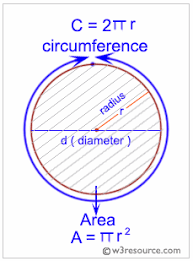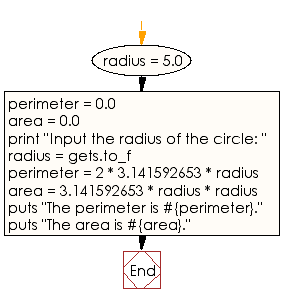﻿ Ruby Basic exercises: Compute the parameter and area - w3resource# Ruby Basic Exercises: Compute the parameter and area

## Ruby Basic: Exercise-4 with Solution

Write a Ruby program which accept the radius of a circle from the user and compute the parameter and area.Ruby Code:

``````radius = 5.0
perimeter = 0.0
area = 0.0
print "Input the radius of the circle: "
perimeter = 2 * 3.141592653 * radius
puts "The perimeter is #{perimeter}."
puts "The area is #{area}."
``````

Output:

```Input the radius of the circle: The perimeter is 31.41592653.
The area is 78.539816325.
```

Flowchart:Ruby Code Editor: